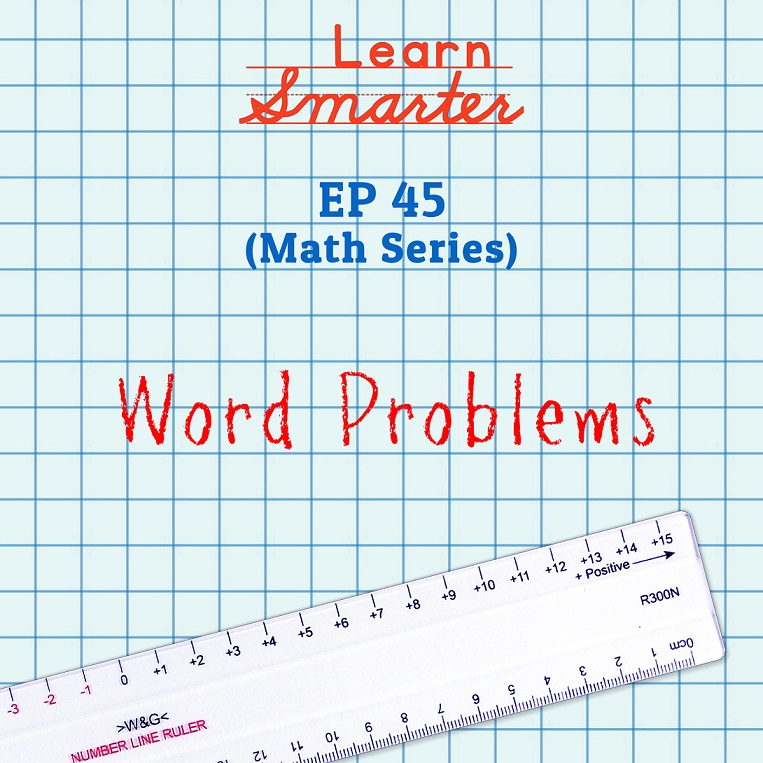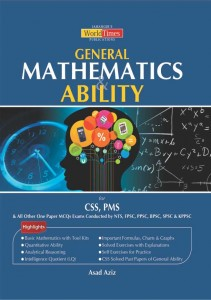Breaking News

# Word Problems# Word Problems

Q1. Two angles are complementary. If one of the angles is double the other angle, find the two angles.

Ans: 30° and 60°

Q2. Two angles are complementary. If one angle is two times the sum of other angle and 3, find the two angles.

Ans: 62° and 28°

Q3. The measure of an angle is ¾ of 60°. What is the measure of the complementary angle?

Ans: 45°

Q4. Two angles are supplementary. If one angle is 36° less than twice of the other angle, find the two angles.

Ans: 108° and 72°

Q5. An angle and its one-half are complementary. Find the angle.

Ans: 60°.

Q6. Two angles are supplementary. If 5 times of one angle is 10 times of the other angle. Find the two angles.

Ans: 60° and 120°.

Q7. The measure of the supplement of angle A is 40 degrees larger than twice the measure of the complement of angle of A. What is the sum in degrees, of the measures of the supplement and complement of angle A?

Ans: 190°

Q8. Twice the complement of an angle is 24 degrees less than its supplement. What is the measure of the angle?

Ans: 24 degrees°

Q9. Two angles are supplementary. If the ratio of the measure of the smaller angle to that of the larger angle is 5:7, what is the measure of the smaller angle?

Ans: 75°.

Q10. The measure of supplement of an angle is equal to the twice the measure of the angle. What is the measure in degrees, of the compliment of the angle?

Ans: 30°Q11. If 4 times the sum of an angle and 5 is 32, find the type of the angle.

Ans: Obtuse angle

Q12. If the sum of 5 times of an angle and 2 is 1222, find the type of the angle.

Ans: Reflex angle

Q13. If the sum of 5 times of an angle and 2 is 1222, find the type of the angle.

Ans: Right angle

Q14. If 7 times the difference between 3 times of an angle and 5 is 3745, find the type of the angle.

Ans: Straight angle

Q15. If 2 times the difference between 9 times of angle and 15 is 6450, find the type of the angle.

Ans: Full angle

Q16. In a triangle, the ratio between the first and second angle is 1:2 and the third angle is 72. Find the first and second angle of the triangle.

Ans: 36° and 72°

Q17. In a triangle, if the second angle is 3 times the sum of the first angle and 3 and the third angle is the sum of 2 times the first angle and 3, find the three angles of the triangle.

Ans: 28°, 93° and 59°

Q18. In a right triangle, apart from the right angle, the other two angles are x + 1 and 2x + 5. Find the angles of the triangle.

Ans: Hence 90°, 29° and 61°

Q19. In a triangle, if the second angle is 2 times the first angle and the third angle is 3 times the first angle, find the angles of the triangle.

Ans: 30°, 60° and 90°

Q20. If 3 consecutive positive integers be the angles of a triangle, then find the three angles of the triangle.

Ans: 59°, 60° and 61°

Box

Did you know?

Complementary angles are two angles with a sum of 90∘. A common case is when they form a right angle. Supplementary angles, on the other hand, are two angles with a sum of 180∘. A common case is when they lie on the same side of a straight line.